# Difference between revisions of "Smallest quadratic nonresidue is less than square root plus one"

## Statement

Supposeis an odd prime. Supposeis the smallest positive integer less thanthat is a quadratic nonresidue modulo. Then:.

Note that we need theadditive correction term. For instance, in the cases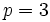and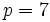, the smallest quadratic nonresidues (andrespectively) are greater than the squareroot.

## Related facts

### Other related facts and conjectures

• Extended Riemann hypothesis: A stronger form of the Riemann hypothesis that is equivalent to the statement that the smallest quadratic nonresidue modulo, for any odd prime, is less than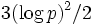, wherehere is the natural logarithm.
• Artin's conjecture on primitive roots: This is a closely related conjecture that states that every integer that is notor a perfect square occurs as a primitive root for infinitely many primes.

## Proof

Given: An odd prime,is the smallest positive integer that is a quadratic nonresidue modulo.

To prove:.

Proof: Letbe the smallest positive integer such that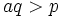, and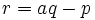. Sinceis prime,, so. Further, by the multiplicativity of Legendre symbols: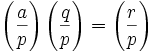.

Sinceis a quadratic nonresidue mod, the first term is, so at least one ofandis a nonresidue. Sinceandis the least nonresidue by assumption,must be a quadratic residue, forcingto be a quadratic nonresidue, and hence,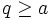. Since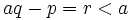, we get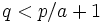. In particular, since, from which we can deduce that.